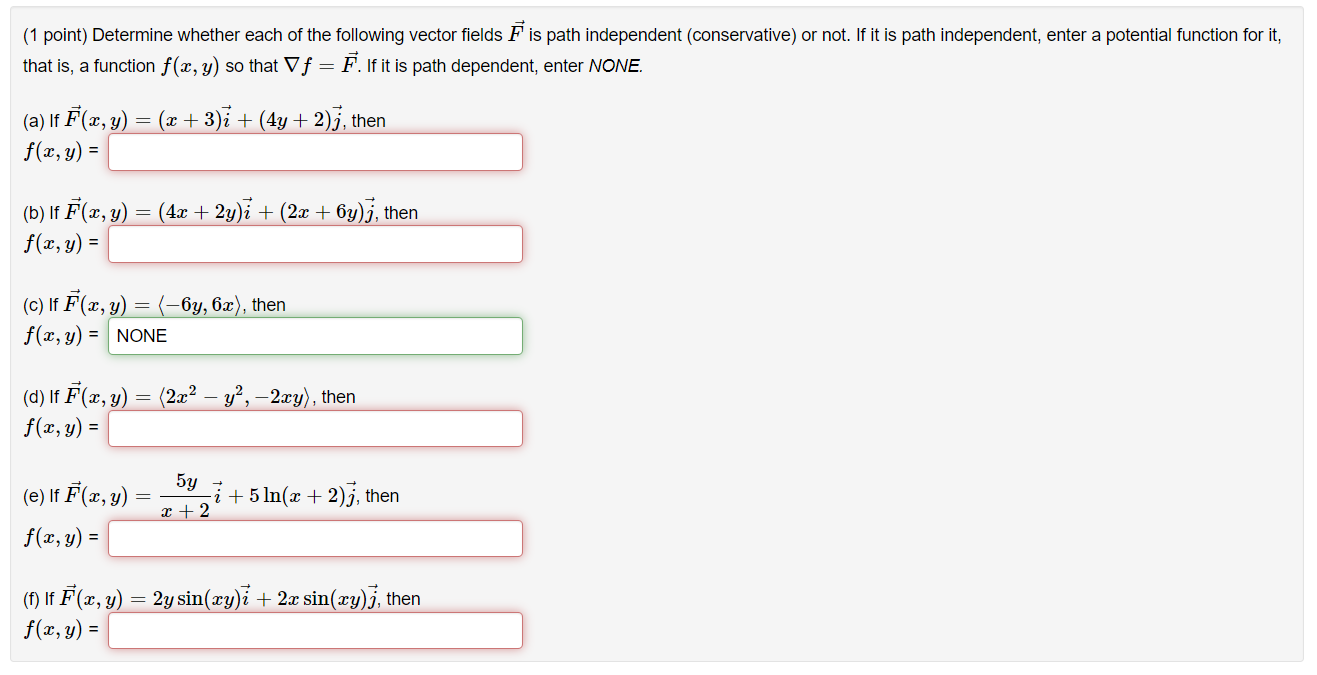### Create an Account

Already have account?

### Forgot Your Password ?

Home / Questions / (1 point) Determine whether each of the following vector fields F is path independent (con...

# (1 point) Determine whether each of the following vector fields F is path independent (conservative) or not. If it is path independent, enter a potential function for it, that is, a function f(x, y) s

(1 point) Determine whether each of the following vector fields F is path independent (conservative) or not. If it is path independent, enter a potential function for it, that is, a function f(x, y) so that Vf Ē. If it is path dependent, enter NONE. + (a) If F(x, y) = (x + 3)i + (4y + 2);, then f(x,y) = (b) If F(x, y) = (4x + 2y)i + (2x + 6y)), then f(x, y) = (C) If F(x, y) = (-6y, 6x), then f(x, y) = NONE (d) If F(x, y) = (2x2 – y’, –2cy), then f(x, y) = 5y i + 5 In(x + 2);, then (e) If F(x, y) = f(x, y) = x + 2 (1) If F(x, y) = 2y sin(xy)ī + 2x sin(xy), then f(x, y) =Apr 14 2021 View more View Less

#### Answer (Solved)Subscribe To Get Solution